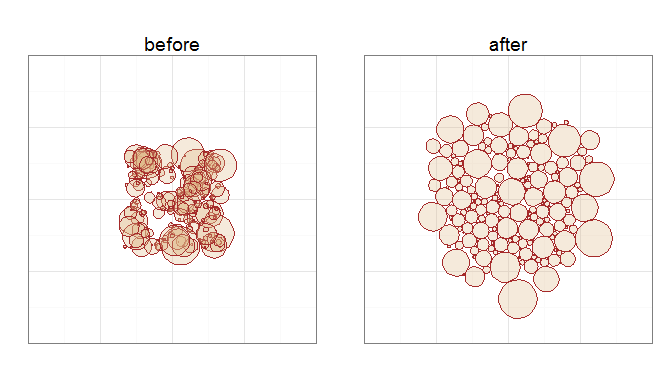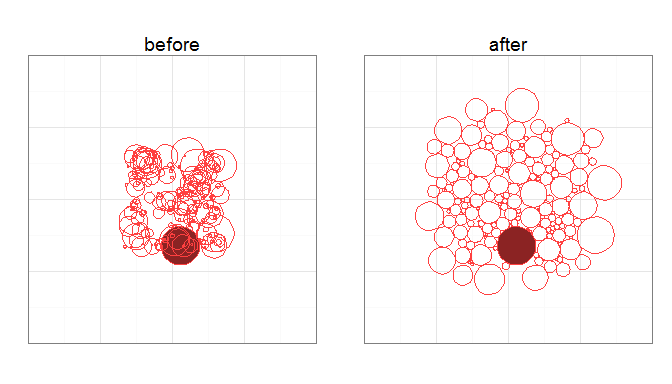In this example we will:

• create a set of randomly positioned, overlapping circles of various sizes;
• use the circleLayout function to find a non-overlapping arrangement;
• use ggplot to draw the before and after arrangements.

Firstly lets create some random circles, positioned within the central portion of a bounding square, with smaller circles being more common than larger ones.

ncircles <- 200
limits <- c(-50, 50)
inset <- diff(limits) / 3
rmax <- 20

xyr <- data.frame(
x = runif(ncircles, min(limits) + inset, max(limits) - inset),
y = runif(ncircles, min(limits) + inset, max(limits) - inset),
r = rbeta(ncircles, 1, 10) * rmax)

Next, we use the circleLayout function to try to find a non-overlapping arragement, allowing the circles to occupy any part of the bounding square. The returned value is a list with elements for the layout and the number of iterations performed.

library(packcircles)

res <- circleLayout(xyr, limits, limits, maxiter = 1000)
cat(res$niter, "iterations performed") ## 471 iterations performed Now we can use ggplot to draw the before and after layouts. library(ggplot2) library(gridExtra) ## Loading required package: grid ## plot data for the before layout dat.before <- circlePlotData(xyr) ## plot dta for the after layout returned by circleLayout dat.after <- circlePlotData(res$layout)

doPlot <- function(dat, title)
ggplot(dat) +
geom_polygon(aes(x, y, group=id), colour="brown", fill="burlywood", alpha=0.3) +
coord_equal(xlim=limits, ylim=limits) +
theme_bw() +
theme(axis.text=element_blank(),
axis.ticks=element_blank(),
axis.title=element_blank()) +
labs(title=title)

grid.arrange(
doPlot(dat.before, "before"),
doPlot(dat.after, "after"),
nrow=1)## Moving and fixed circles

The circleLayout function accepts an optional weights argument to give extra control over the movement of circles at each iteration of the layout algorithm. The argument takes a numeric vector with values in the range 0-1 inclusive (any values outside this range will be clamped to 0 or 1). A weight of 0 prevents a circle from moving at all while a weight of 1 allows full movement.

As an example, we will make the largest circle in the xyr data.frame static, and colour it differently in the before and after plots:

largest.id <- which(xyr$r == max(xyr$r))

# add a column to the previously generated plot data for the 'before' circles
dat.before$state <- ifelse(dat.before$id == largest.id, "static", "free")

# tweak the plot function to colour circles based on the state column
doPlot <- function(dat, title)
ggplot(dat) +
geom_polygon(aes(x, y, group=id, fill=state), colour="brown1") +
scale_fill_manual(values=c("NA", "brown4")) +
coord_equal(xlim=limits, ylim=limits) +
theme_bw() +
theme(axis.text=element_blank(),
axis.ticks=element_blank(),
axis.title=element_blank(),
legend.position="none") +
labs(title=title)

g.before <- doPlot(dat.before, "before")

# now re-run the layout algorithm with a weights vector to fix the position
# of the largest circle
wts <- rep(1.0, nrow(xyr))
wts[ largest.id ] <- 0.0

res <- circleLayout(xyr, limits, limits, maxiter = 1000, weights=wts)

# finally generate a new plot for the 'after' circles
dat.after <- circlePlotData(res$layout) dat.after$state <- ifelse(dat.after\$id == largest.id, "static", "free")

g.after <- doPlot(dat.after, "after")

grid.arrange(g.before, g.after, nrow=1)